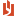## MS Mod 21: Multiple regression analysis – practice problems

 Author Message NEASSupreme BeingGroup: Administrators Posts: 4.2K, Visits: 1.2K MS Module 21: Multiple regression analysis – practice problems(The attached PDF file has better formatting.)Exercise 21.1: Multiple regression A multiple regression analysis with 5 data points and two independent variables X1 and X2 has the following actual values (yi) and fitted values (ŷi):Actual Value    3    2    3    6    11Fitted Value    1    3    5    7    9●    The null hypothesis is H0: β1 = β2 = 0●    The alternative hypothesis is Ha: β1 ≠ 0 or β2 ≠ 0A.    What are the residuals for the five data points?B.    What is the total sum of squares (SST)?C.    What is the error sum of squares (SSE)?D.    What is s2, the least squares estimate for σ2?E.    What is R2?F.    What is the adjusted R2?G.    What is the test statistic value f to test the null hypothesis?H.    What is the p value for this null hypothesis? obs    fitted    actual    residual    SST    SSE#1    1    3    2    4    4#2    3    2    -1    9    1#3    5    3    -2    4    4#4    7    6    -1    1    1#5    9    11    2    36    4avg    5    5    0    54    14Part A: Each residual is the actual value minus the fitted value. Illustration: For the first observation, the residual is 3 – 1 = 2.Part B: The total sum of squares is the sum of squared deviations from the mean. The average of the actual values is (3 + 2 + 3 + 6 + 11) / 5 = 25 / 5 = 5. The squared deviation for the first observation is (3 – 5)2 = 4. The sum of the squared deviations is 54.Part C: The error sum of squares SSE is like the total sum of squares SST except that it uses the residuals instead of the actual values. The average residual is zero, so we take the sum of squared residuals = 14.Part D: s2, the least squares estimate for σ2, is SSE / degrees of freedom. With 5 data points and three parameters in the multiple regression equation (β0, β1, β2), the degrees of freedom = 5 – 3 = 2, so    s2 = 14 / 2 = 7.Take heed: statisticians differ in their use of the term parameters: ●    Some speak of two slope parameters (β1, β2) and n – (k + 1) degrees of freedom (= textbook’s usage). ●    Some speak of three slope + intercept parameters (β0, β1, β2) and n – k degrees of freedom. Part E: R2 = 1 – SSE / SST = 1 – 14 / 54 = 0.74074Part F: The adjusted R2 (page 686 in second edition; page 672 in first edition) = 1 – MSE / MST = 1 – [ SSE / (n - (k + 1) ] / [ SST / (n - 1) ] = 1 – (n-1)/(n-(k+1)) × SSE/SST =    1 - (5 - 1) / (5 - 2 - 1) × 14 / 54 = 0.48148Part G: The test statistic f = [ R2 / k ] / [ (1 – R2) / (n - (k + 1) ) ] =    (0.74074 / 2) / ( (1 - 0.74074) / (5 - 2 - 1) ) = 2.85713Part H: The p value for an f value with 2 degrees of freedom in the numerator and 2 degrees of freedom in the denominator is 0.25926. AttachmentsMS Module 21 Multiple regression analysis – practice problems df.pdf (147 views, 57.00 KB) Edited 2 Years Ago by NEAS
##### Merge Selected
Merge into selected topic...

Merge into merge target...

Merge into a specific topic ID...